##### Geometry Workbook For DummiesThe following two theorems — If sides, then angles and If angles, then sides — are based on a simple idea about isosceles triangles that happens to work in both directions: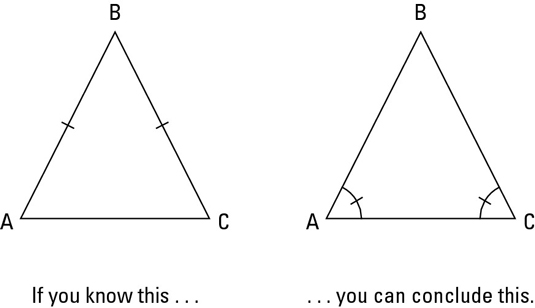• If sides, then angles: If two sides of a triangle are congruent, then the angles opposite those sides are congruent. The above figure shows you how this works.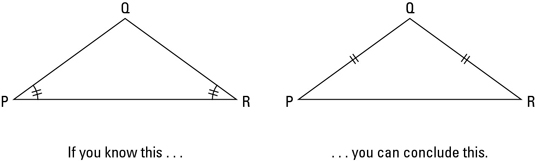• If angles, then sides: If two angles of a triangle are congruent, then the sides opposite those angles are congruent. The above figure shows an example of this.

Look for isosceles triangles. The two angle-side theorems are critical for solving many proofs, so when you start doing a proof, look at the diagram and identify all triangles that look like they’re isosceles. Then make a mental note that you may have to use one of the angle-side theorems for one or more of the isosceles triangles. These theorems are incredibly easy to use if you spot all the isosceles triangles (which shouldn’t be too hard). But if you fail to notice the isosceles triangles, the proof may become impossible. And note that your goal here is to spot single isosceles triangles because unlike SSS (side-side-side), SAS (side-angle-side), and ASA (angle-side-angle), the isosceles-triangle theorems do not involve pairs of triangles.

Here’s a proof. Try to work through a game plan and/or a formal proof on your own before reading the ones presented here.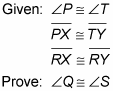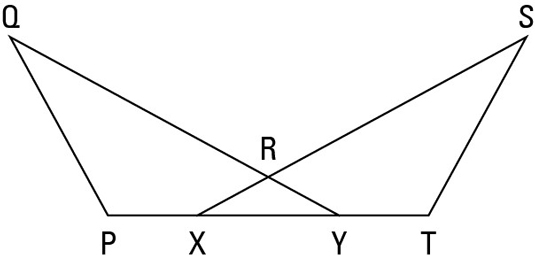Here’s a game plan:

• Check the proof diagram for isosceles triangles and pairs of congruent triangles. This proof’s diagram has an isosceles triangle, which is a huge hint that you’ll likely use one of the isosceles triangle theorems. You also have a pair of triangles that look congruent (the overlapping ones), which is another huge hint that you’ll want to show that they’re congruent.

• Think about how to finish the proof with a triangle congruence theorem and CPCTC (Corresponding Parts of Congruent Triangles are Congruent). You’re given the sides of the isosceles triangle, so from that you can get congruent angles. You’re also given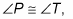so that gives you a second pair of congruent angles. If you can get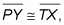you’d have ASA. And you can get that by adding line segment XY to the given congruent segments, PX and TY. You finish with CPCTC.

Check out the formal proof:

Statement 1: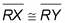Reason for statement 1: Given.

Statement 2: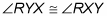Reason for statement 2: If two sides of a triangle are congruent, then the angles opposite those sides are congruent.

Statement 3: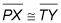Reason for statement 3: Given.

Statement 4: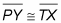Reason for statement 4: If a segment is added to two congruent segments, then the sums are congruent.

Statement 5: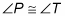Reason for statement 5: Given.

Statement 6: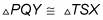Reason for statement 6: ASA (using lines 2, 4, and 5).

Statement 7: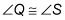Reason for statement 7: CPCTC.